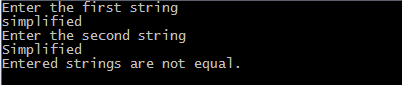## Print this Job Post# c program to compare two strings

## C program to compare two strings using strcmp

#include <stdio.h>
#include <string.h>
void main()
{
char a, b;
clrscr();
printf("Enter the first string\n");
gets(a);
printf("Enter the second string\n");
gets(b);
if( strcmp(a,b) == 0 )
printf("Entered strings are equal.\n");
else
printf("Entered strings are not equal.\n");
getch();
}

## Output of program:## C program to compare two strings without using strcmp

Here we create our own function to compare strings.
int compare(char a[], char b[])
{
int c = 0;
while( a[c] == b[c] )
{
if( a[c] == '\0' || b[c] == '\0' )
break;
c++;
}
if( a[c] == '\0' && b[c] == '\0' )
return 0;
else
return -1;
}

## C program to compare two strings using pointers

In this method we will make our own function to perform string comparison, we will use character pointers in our function to manipulate string.
#include<stdio.h>
#include<conio.h>
int compare_string(char*, char*);
void main()
{
char first, second, result;
clrscr();
printf("Enter first string\n");
gets(first);
printf("Enter second string\n");
gets(second);
result = compare_string(first, second);
if ( result == 0 )
printf("Both strings are same.\n");
else
printf("Entered strings are not equal.\n");
getch();
}
int compare_string(char *first, char *second)
{
while(*first==*second)
{
if ( *first == '\0' || *second == '\0' )
break;
first++;
second++;
}
if( *first == '\0' && *second == '\0' )
return 0;
else
return -1;
}